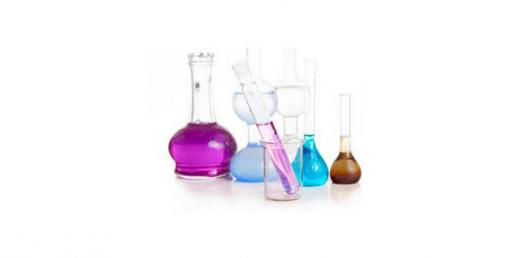# Identifying Chemicals And Substances

15 Questions | Total Attempts: 4372SettingsChemistry Quiz 1.

• 1.
What characteristic, or property, is shared by all forms of matter?
• A.

Color

• B.

Oxygen

• C.

Mass

• D.

Chemical make-up

• 2.
Which of the following represents a mixture?
• A.

A piece of copper wire

• B.

The oxygen gas in a pressurized tank

• C.

A slice of mushroom pizza

• D.

• 3.
What property of matter is being illustrated in this drawing?
• A.

Ability to conduct electricity

• B.

Magnetism

• C.

Malleability

• D.

Ability to conduct heat

• 4.
What must be known in order to calculate density?
• A.

Mass and solubility

• B.

Mass and length

• C.

Mass and color

• D.

Mass and volume

• 5.
What does the word malleable mean?
• A.

The substance can be pounded into a thin sheet.

• B.

The substance can dissolve in water.

• C.

The substance can conduct electricity.

• D.

The substance has a high density.

• 6.
Which of the following is an example of a solution?
• A.

Melting ice

• B.

Sand and water

• C.

Boiling water

• D.

Alcohol in water

• 7.
Which of the following is an example of a chemical change?
• A.

Water evaporating

• B.

Wood burning

• C.

Ice cream melting

• D.

Face blushing

• 8.
A substance that allows electricity and/or heat to pass through it is __________.
• A.

Magnetic

• B.

A conductor

• C.

An insulator

• D.

A catalyst

• 9.
Which is true about a chemical change in matter?
• A.

A chemical change causes a change in mass

• B.

Substances never change phase as a part of a chemical change.

• C.

Matter is destroyed during a chemical change

• D.

Atoms are rearranged during a chemical change.

• 10.
Which of the following is a homogeneous mixture?
• A.

• B.

A lava lamp

• C.

Apple juice

• 11.
The process of water changing from a liquid to a gas.
• A.

Condensation

• B.

Evaporation

• C.

Precipitation

• D.

Melting point

• 12.
Which of the following is an example of a physical property?
• A.

Flammability

• B.

Reactivity

• C.

Color

• 13.
The ability to dissolve is called ___________.
• A.

Solubility

• B.

Filtration

• C.

Insoluble

• D.

Magnetism

• 14.
A __________ is a substance that cannot be broken down into a simpler substance. These are organized on the periodic table.
• A.

Compound

• B.

Mixture

• C.

Element

• D.

Solution

• 15.
The amount of salt in a a substance is called ______________.
• A.

Salinity

• B.

Chemical

• C.

Compound

• D.

Conductivity

Related TopicsBack to top